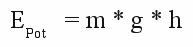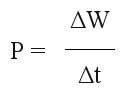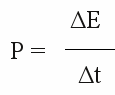# Work, energy and power

## Work

Mechanical work is done whenever a force is moving an object trough a distance:

[2.10]Where is:
W - work, F - force pointing along distance, s - distance

## Energy

Work is transforming energy into another form. There are two types of mechanical energy:
1.) Potential energy:

[2.11]Where is:
EPot - Potential energy, m - mass of the lifted object, g - gravitational acceleration, h - height difference

2.) Kinetic energy:

[2.12]Where is:
EKin - kinetic energy, m - mass of the object, v - speed

## Strictly speaking

one object doesn't attract another one, but rather the force between the objects affects both of them. Looking at a car and the earth, you will find out that the mass and therewith their gravitational fields differ significantly. The mass of the earth is about 5.973.600.000.000.000.000.000.000 kg, a compact car less than 1.000 kg. Accordingly you push the earth just a little distance away while lifting the car. The gravitational field between earth and car is not constant, but the difference of the gravitational acceleration will be neglected in the following. It is 10,82m/s2 at sea level and 9,52m/s2 100m above that. We won't design a car for the "Pikes Peak International Hill Climb", whereat 1.439m of height difference have to be crested, but an everyday car having rarely to climb more than 100m even at the hills of Westerwald and Taunus.
As mentioned at the chapter about velocities and subsequently, movement is relative. Strictly speaking you push the earth away while accelerating your car, but the enormous mass difference permits to neglect the earth's change of movement.

## Power

Power is the rate at which work is performed or energy is converted:

[2.13a]or
[2.13b]Where is:
P - power, ΔW - work interval, Δt - time interval, ΔE - energy interval